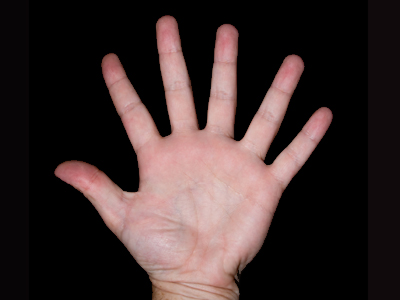6 is the digit in the ten thousands' place in 68,127.

# Place Value 6

This Math quiz is called 'Place Value 6' and it has been written by teachers to help you if you are studying the subject at elementary school. Playing educational quizzes is an enjoyable way to learn if you are in the 3rd, 4th or 5th grade - aged 8 to 11.

It costs only \$12.50 per month to play this quiz and over 3,500 others that help you with your school work. You can subscribe on the page at Join Us

Place value is about the position of a number. In this quiz you will get the chance to revise place values in whole numbers < 100,000. Use this information to help you find a digit's place value:

Reading the number from right to left: the first digit is in the units' place; the second digit is in the tens' place; the third digit is in the hundreds' place; the fourth digit is in the thousands' place; the fifth digit is in the ten thousands' place.

For example, in 83,659 the 9 is in the units' place; the 5 is in the tens' place; the 6 is in the hundreds' place; the 3 is in the thousands' place; the 8 is in the ten thousands' place.

1.
What is the place value of 5 in 63,597?
The units' place
The thousands' place
The hundreds' place
The tens' place
Reading the number from right to left: 5 is in the hundreds' place
2.
What is the digit in the tens' place in 63,507?
0
5
7
6
Reading the number from right to left: 0 is in the tens' place
3.
What is the place value of 8 in 83,537?
The tens' place
The hundreds' place
The ten thousands' place
The thousands' place
Reading the number from right to left: 8 is in the ten thousands' place
4.
What is the digit in the thousands' place in 43,566?
5
4
3
6
Reading the number from right to left: 3 is in the thousands' place
5.
What is the digit in the units' place in 88,329?
3
2
8
9
Reading the number from right to left: 5 is in the units' place
6.
What is the place value of 3 in 63,400?
The hundreds' place
The thousands' place
The tens' place
The ten thousands' place
Reading the number from right to left: 3 is in the thousands' place
7.
What is the place value of 2 in 92,347?
The hundreds' place
The thousands' place
The ten thousands' place
The tens' place
Reading the number from right to left: 2 is in the thousands' place
8.
What is the digit in the hundreds' place in 92,067?
9
6
7
0
Reading the number from right to left: 0 is in the hundreds' place
9.
What is the digit in the ten thousands' place in 68,127?
6
8
2
7
Reading the number from right to left: 6 is in the ten thousands' place
10.
What is the place value of 1 in 64,601?
The units' place
The hundreds' place
The tens' place
The thousands' place
Reading the number from right to left: 1 is in the units' place
Author:  Frank Evans